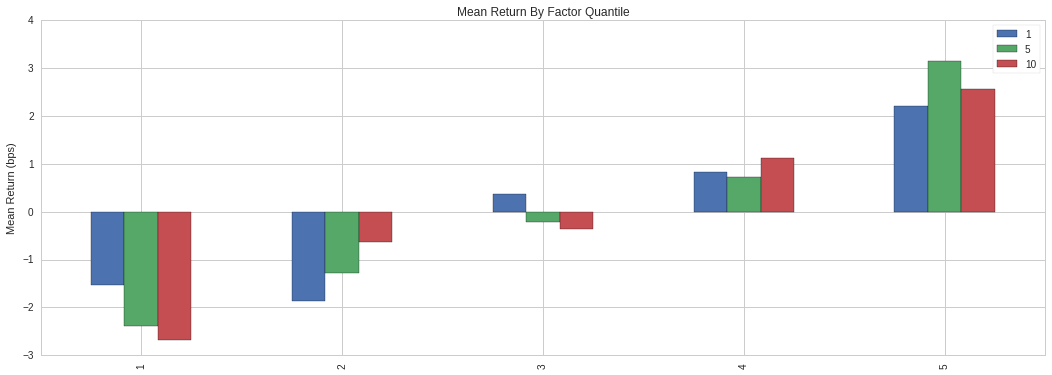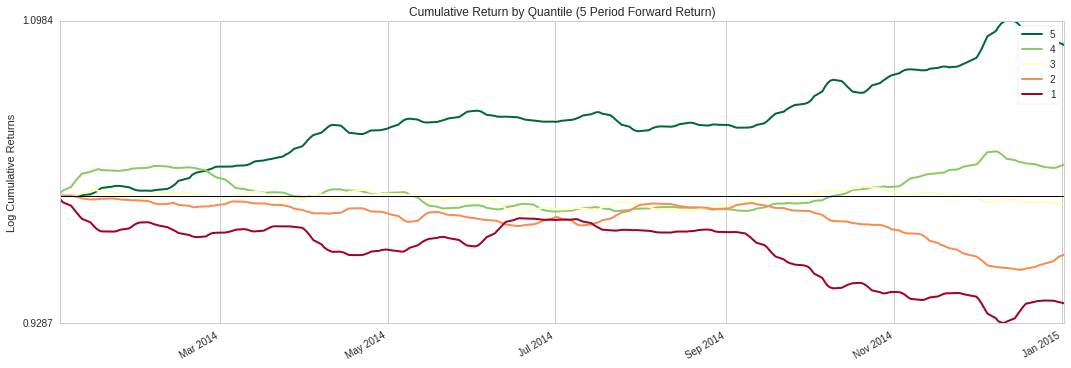Notebook
In :
from quantopian.pipeline import Pipeline
from quantopian.research import run_pipeline
from quantopian.pipeline.factors import CustomFactor
from quantopian.pipeline.filters import Q1500US
from quantopian.pipeline.classifiers.morningstar import Sector
from quantopian.pipeline.data.builtin import USEquityPricing
from quantopian.pipeline.data.psychsignal import stocktwits
import alphalens as al
import numpy as np


## Psychsignal - StockTwits Trader Mood & Optimize API (Long/Short)Â¶

Psychsignal's StockTwits Trader Mood analyzes trader's messages posted on StockTwits, and provides a measure of bull/bear intensity for securities based on message sentiment.

In this notebook, we will construct a couple of pipeline factors based on this dataset and analyze them using Alphalens to determine if the can effectively predict returns. After, we will develop an algorithm based on the results of our analysis.

Psychsignal's factors used in this notebook:

bull_minus_bear - subtracts the bearish intesity from the bullish intensity [BULL - BEAR] to provide an immediate net score. bull_scored_messages - total count of bullish sentiment messages scored by PsychSignal's algorithm bear_scored_messages - total count of bearish sentiment messages scored by PsychSignal's algorithm

## Defining our FactorsÂ¶

The following custom factors calculate the average [BULL - BEAR] intensity over the past 3 days, and the average number of messages on a 30 day period. We will use [BULL - BEAR] intensity to rank securities based on trader mood, and we will only consider the top 1000 securities by average number of messages over a 30 day period.

In :
class BullBearIntensity(CustomFactor):
"""
Baseline PsychSignal Factor
"""
inputs = [stocktwits.bull_minus_bear]
window_length = 3

def compute(self, today, assets, out, bull_minus_bear):
np.nanmean(bull_minus_bear, axis=0, out=out)

class PsychSignalMessages(CustomFactor):
"""
Created to rank each security by message coverage
"""
inputs = [stocktwits.bull_scored_messages, stocktwits.bear_scored_messages]
window_length = 30

def compute(self, today, assets, out, bull_msgs, bear_msgs):
np.nanmean(bull_msgs + bear_msgs, axis=0, out=out)


First, we need to run our pipeline over the period of time that we want to analyze. We will look at a 1 year period, between 2014-01-01 and 2015-01-01.

In :
# Run pipeline over 1 year period

def make_pipeline():
"""
Create our pipeline.
"""
message_rank = PsychSignalMessages().rank(ascending=False)

universe = Q1500US() & (1000 > message_rank)
sector = Sector()
sentiment = BullBearIntensity().rank()

return Pipeline(
columns={
'sentiment': sentiment,
'sector': sector
},
screen = universe
)

results = run_pipeline(make_pipeline(), '2014-01-01', '2015-01-01')
results.fillna(value=0);

In :
results.head(5)

Out:
sector sentiment
2014-01-02 00:00:00+00:00 Equity(2 [ARNC]) 101 4779.0
Equity(24 [AAPL]) 311 719.0
Equity(88 [ACI]) 101 3959.0

Next, we need pricing data for securities that were present in our trading universe over our 1 year period

In :
# Get list of unique assets present at any given time
asset_list = results.index.levels.unique()

# Get pricing data over 1 year period + an extra month of out-of-sample
prices = get_pricing(asset_list, start_date='2014-01-01', end_date='2015-02-01', fields='price')

In :
prices.head(5)

Out:
Equity(2 [ARNC]) Equity(24 [AAPL]) Equity(62 [ABT]) Equity(67 [ADSK]) Equity(76 [TAP]) Equity(88 [ACI]) Equity(110 [ACXM]) Equity(114 [ADBE]) Equity(122 [ADI]) Equity(128 [ADM]) ... Equity(47382 [LOCO]) Equity(47415 [SYF]) Equity(47430 [MBLY]) Equity(47752 [CDK]) Equity(47777 [CFG]) Equity(47779 [CYBR]) Equity(47888 [FCAU]) Equity(48091 [VA]) Equity(48104 [PGRE]) Equity(48220 [LC])
2014-01-02 00:00:00+00:00 10.440 77.405 37.206 49.25 54.012 4.639 36.58 59.28 47.901 42.117 ... NaN NaN NaN NaN NaN NaN NaN NaN NaN NaN
2014-01-03 00:00:00+00:00 10.480 75.700 37.585 48.89 53.807 4.409 36.24 59.16 48.222 42.293 ... NaN NaN NaN NaN NaN NaN NaN NaN NaN NaN
2014-01-06 00:00:00+00:00 10.441 76.107 38.091 48.53 53.601 4.419 35.96 58.10 47.950 42.401 ... NaN NaN NaN NaN NaN NaN NaN NaN NaN NaN
2014-01-07 00:00:00+00:00 10.461 75.559 37.799 49.66 54.149 4.320 36.36 58.96 48.193 41.970 ... NaN NaN NaN NaN NaN NaN NaN NaN NaN NaN
2014-01-08 00:00:00+00:00 10.728 76.043 38.140 50.25 54.012 4.150 36.25 58.89 48.319 41.490 ... NaN NaN NaN NaN NaN NaN NaN NaN NaN NaN

5 rows × 1162 columns

Alphalens allows us to group our assets by sector, so we will use Morningstar's sector map and the sector codes returned by our pipeline.

In :
# Extract Sector mappings from pipeline output
sectors = results['sector']

In :
# Instantiate a sector code to sector name map.
# We will provide this to Alphalens as sector labels
sector_names = Sector.SECTOR_NAMES
sector_names[Sector.missing_value] = 'None'


Now we will use Alphalens to get the forward returns of our factor for perios of 1, 5 and 10 holding days. Alphalens does not take into account commissions nor slippage, it just gives us a rough idea of what the returns would have been if we happened to hold a position for a given asset during the holding period.

In :
factor_data = al.utils.get_clean_factor_and_forward_returns(factor=results['sentiment'],
prices=prices,
groupby=sectors,
groupby_labels=sector_names,
periods=(1,5,10))

In :
factor_data.head(5)

Out:
1 5 10 factor group factor_quantile
date asset
2014-01-02 00:00:00+00:00 Equity(2 [ARNC]) 0.003831 0.015230 0.048467 4779.0 BASIC_MATERIALS 4
Equity(24 [AAPL]) -0.022027 -0.030231 0.001809 719.0 TECHNOLOGY 1
Equity(67 [ADSK]) -0.007310 0.037157 0.088122 4050.0 TECHNOLOGY 2
Equity(88 [ACI]) -0.049580 -0.101099 -0.053891 3959.0 BASIC_MATERIALS 2
Equity(114 [ADBE]) -0.002024 -0.003036 0.039305 3947.0 TECHNOLOGY 2

Notice factor_data also includes a factor_quantile column which classifies securities based on their returns for a given date.

Let's use Alphalens to compute mean returns by quantile, and plot the corresponding buckets. If our factor is a good predictor of returns, higher quantiles should have higher returns, and lower quantiles should have lower returns. This will help us build our Long/Short strategy later.

In :
mean_return_by_q, std_err_by_q = al.performance.mean_return_by_quantile(factor_data,
by_group=False)

In :
al.plotting.plot_quantile_returns_bar(mean_return_by_q.apply(al.utils.rate_of_return, axis=0));This confirms our pipeline factor is a pretty good predictor of returns.

This plot can also give us a rough idea of what would be a good turnover frequency for our strategy. A 5 day holding period seems to be a good choice here since it has the highest returns for quantile 5, and decently low returns for quantile 1.

Let's now look at the returns over time by quantile for a 5 day holding period.

In :
mean_return_by_q_daily, std_err_by_q_daily = al.performance.mean_return_by_quantile(factor_data,
by_date=True)

In :
al.plotting.plot_cumulative_returns_by_quantile(mean_return_by_q_daily, period=5);We can see returns for quantile 5 consistently increase. This is good since it represents the return stream of securities with highest alpha value. Returns in quantile 1 have an up trend bewtween mid May, 2014 and late July, 2014. This might have a negative effect on our strategy, so it would be interesting to see how our strategy behaves over that period.

Using what we have learned about our factor using Alphalens, let's build our strategy.